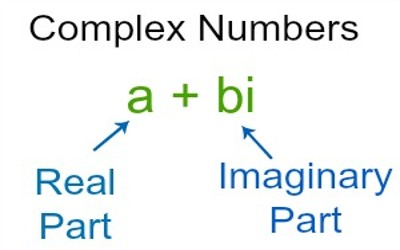Mathematic

# Equality of Complex NumbersEquality of Complex Numbers

A Complex Number is a combination of a Real Number and an Imaginary Number. A complex number is a number that can be expressed in the form a + bi, where a and b are real numbers, and ‘i’ is a solution of the equation x2 = −1, which is called an imaginary number because there is no real number that satisfies this equation.

Here discuss the equality of complex numbers-

Two complex numbers z1 = a + ib and z2 = x + iy are equal if and only if a = x and b = y i.e., Re (z1) = Re (z2) and Im (z1) = Im (z2).

Thus, z1 = z2 ⇔ Re (z1) = Re (z2) and Im (z1) = Im (z2).

For example, if the complex numbers z1 = x + iy and z2 = -5 + 7i are equal, then x = -5 and y = 7.Solved examples on equality of two complex numbers:

1. If z1 = 5 + 2yi and z2 = -x + 6i are equal, find the value of x and y.

Solution:

The given two complex numbers are z1 = 5 + 2yi and z2 = -x + 6i.

We know that, two complex numbers z1 = a + ib and z2 = x + iy are equal if a = x and b = y.

z1 = z2

⇒ 5 + 2yi = -x + 6i

⇒ 5 = -x and 2y = 6

⇒ x = -5 and y = 3

Therefore, the value of x = -5 and the value of y = 3.

1. If a, b are real numbers and 7a + i(3a – b) = 14 – 6i, then find the values of a and b.

Solution:

Given, 7a + i(3a – b) = 14 – 6i

⇒ 7a + i(3a – b) = 14 + i(-6)

Now equating real and imaginary parts on both sides, we have

7a = 14 and 3a – b = -6

⇒ a = 2 and 3 ∙ 2 – b = -6

⇒ a = 2 and 6 – b = -6

⇒ a = 2 and – b = -12

⇒ a = 2 and b = 12

Therefore, the value of a = 2 and the value of b = 12.

Information Source: